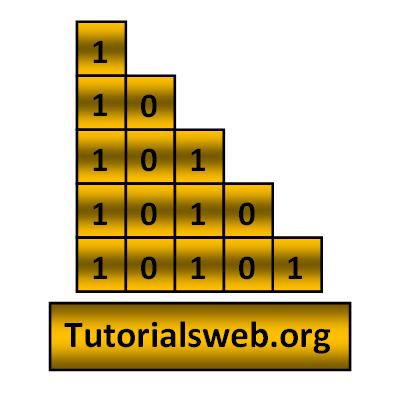## Write "C"  program to print below Number pattern 20:To understand this example, you should have knowledge of the following topics:
·      C programming operators
C Number pattern 20 program using For loop:
```
#include<stdio.h>
int main()
{
int i,j;
for(i=1;i<=5;i++)
{
for(j=1;j<=i;j++)
{
printf("%d",j%2);
}
printf("\n");
}
return 0;
}
```
Output:
`1`
`10`
`101`
`1010`
`10101`
Explanation:
• First two integers "i", "j" are declared of type int.
• Then in the First for loop “i” value is initialized with a value 1.
• Now “i” value is checked with the condition i < 5 that is (1 < 5). So the condition is True.
• Now the loop enters into second for loop and checks the condition j <= i. Where "j = 1". and the condition is True.
• Now prints the " j%2" value . Print up to second for loop condition becomes False.
• when the value of "i"  becomes "6" the program terminates.